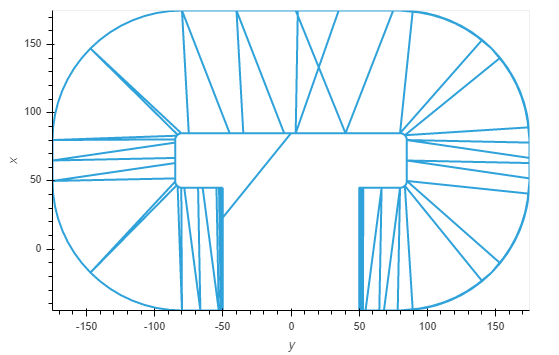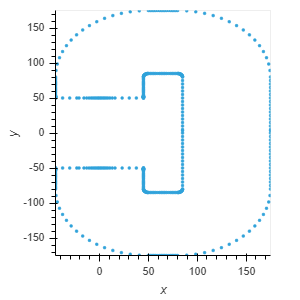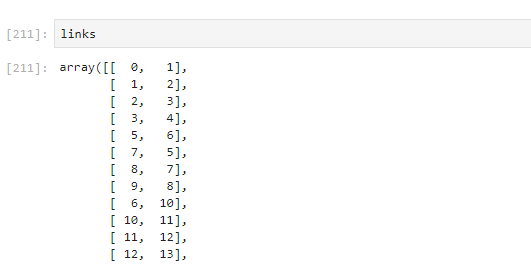# Most efficient way to plot vertex and connections

Hello,
I have 2 arrays the first one is a 2D array with vertices (vertex)
And the second one an array which defines links between vertices (links)
For the moment I use this code to do the plot:

``````hv.Overlay([hv.Path(vertex[link], kdims=['y', 'x']).opts(color='red', line_width=4) for link in links]).opts(...)
``````

It gives me the result I expect:

However since I endup with an overlay with 436 paths the display is slow

Is there a better way to achive this?

Can you simply create a hv.Curve?

No It gives me that :I found the solution:

``````hv.Path(vertex[links].tolist())
``````
1 Like

I’m a bit curious, so do you mind showing a reduced example of what `links` and `vertex` look like?

vertex and links are the intersection of a 3D object with a plane

``````hv.Points(vertex)
``````And links describe the connection of the verticesCool! ThanksSo the `vertex` contains the `(x,y)` points, while `links` contains pairs of indexes which are linked in the `vertex`?

exactly